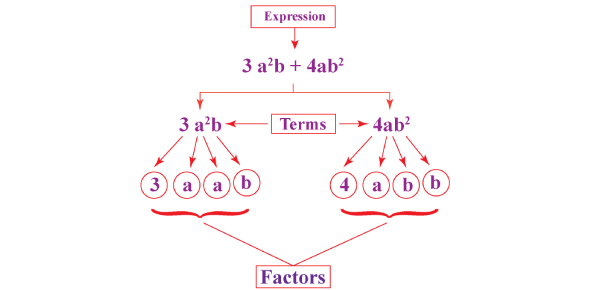# Algebric Equations Factor Quiz

10 Questions | Total Attempts: 174SettingsCreate your own QuizIn Mathematics, factorisation or factoring is defined as breaking or decomposition of entire number, matrix, a polynomial) into a product of another entity, or factors, which when multiplied give the original number or a matrix, etc. Learn how to factor algebric equations in this quiz!

• 1.
Find the factors for the following equation: -6x2 - 3x + 6
• A.

7 and 21

• B.

6 and 18

• C.

2 and 4

• 2.
Find the factors for the following equation: x2 - 4x - 60 **Enter your answers with no spaces and with the smaller factor first. EX: (x-2)(x+1)
• 3.
Find a, b, and c for the following equation: x+ 7x + 12 **Enter your answers starting with a separated with commas and with no spaces unless after the comma. EX: a=1, b=2, c=3
• 4.
Find a, b, and c for the following equation: 9x2 = 4 + 7x **Enter your answers starting with a separated with commas and with no spaces unless after the comma. EX: a=1, b=2, c=3
• 5.
Find the factors for the following equation: x2 - 11x + 24 **Enter your answers with no spaces and with the smaller factor first. EX: (x-2)(x+1)
• 6.
Find the factors for the following equation: 6x2 - 5x - 4 **Enter your answers with no spaces and with the smaller factor first. EX: (x-2)(x+1)
• 7.
Find the factors for the following equation: 6x2 + 7x - 49 **Enter your answers with no spaces and with the smaller factor first. EX: (x-2)(x+1)
• 8.
Find the factors of the following equation: 2x2 + 3x - 20
• A.

Option 1

• B.

Option 2

• C.

Option 3

• 9.
2 + 2x - 5 = 0. Solve for x.
• A.

2

• B.

5

• C.

0

• D.

1.5

• 10.
If 1/x is infinity, what is x?
• A.

0

• B.

1

• C.

1/2

• D.

None of the above

Related TopicsBack to top
×

Wait!
Here's an interesting quiz for you.